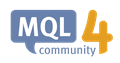# How to Count Total Number & Separate New Line the Label?532

Hi guys, I want to count the total number &  separate new line from the multiple of label (string) that have same value. Example: Sun Opposition with Mercury & Venus Opposition with Mars.
At the moment, my code use OBJPROP_YDISTANCE & plus the range to separate the lines.
So how to separate the same label, in the example is Opposition?

Here's the code:

```void Print_PriceAspectHover(string linename, int bar, double rangesunmoon, double longitudesun, double longitudemercury, double longitudepastmercury, double longitudevenus, double longitudepastvenus, double longitudemars, double longitudepastmars)
{
string initiateline = linename + " ";
string majorname=StringConcatenate(LabelID, "-", initiateline);

int y=1;

string sCommentOpposition = "";

if(ObjectFind(majorname) != 0)
{
int obj_total = ObjectsTotal();
string objectname, objdescription;
int obj_type;
int counter = 0;

for(int i=0; i<obj_total; i++)
{

if (Show_Opposition==true)
{
if (aspecthargasun>=180.0 && aspecthargasun<=180.0)
{
if (Show_Sun==true)
{
sCommentOpposition = planetname + " Opposition with " + planetnature;
}
}
if (aspecthargamercury>=180.0 && aspecthargamercury<=180.0)
{
if (Show_Mercury==true)
{
sCommentOpposition = planetname + " Opposition with " + planetnature;
}
}
if (aspecthargavenus>=180.0 && aspecthargavenus<=180.0)
{
if (Show_Venus==true)
{
sCommentOpposition = planetname + " Opposition with " + planetnature;
}
}
if (aspecthargamars>=180.0 && aspecthargamars<=180.0)
{
if (Show_Mars==true)
{
sCommentOpposition = planetname + " Opposition with " + planetnature;
}
}
}

if (Show_Square==true)
{
if (aspecthargasun>=90.0 && aspecthargasun<=90.0)
{
if (Show_Sun==true)
{
sCommentSquare = planetname + " Square with " + planetnature;
}
}
if (aspecthargamercury>=90.0 && aspecthargamercury<=90.0)
{
if (Show_Mercury==true)
{
sCommentSquare = planetname + " Square with " + planetnature;
}
}
if (aspecthargavenus>=90.0 && aspecthargavenus<=90.0)
{
if (Show_Venus==true)
{
sCommentSquare = planetname + " Square with " + planetnature;
}
}
if (aspecthargamars>=90.0 && aspecthargamars<=90.0)
{
if (Show_Mars==true)
{
sCommentSquare = planetname + " Square with " + planetnature;
}
}
}

if (Show_SemiSquare==true)
{
if (aspecthargasun>=45.0 && aspecthargasun<=45.0)
{
if (Show_Sun==true)
{
sCommentSemiSquare = planetname + " SemiSquare with " + planetnature;
}
}
if (aspecthargamercury>=45.0 && aspecthargamercury<=45.0)
{
if (Show_Mercury==true)
{
sCommentSemiSquare = planetname + " SemiSquare with " + planetnature;
}
}
if (aspecthargavenus>=45.0 && aspecthargavenus<=45.0)
{
if (Show_Venus==true)
{
sCommentSemiSquare = planetname + " SemiSquare with " + planetnature;
}
}
if (aspecthargamars>=45.0 && aspecthargamars<=45.0)
{
if (Show_Mars==true)
{
sCommentSemiSquare = planetname + " SemiSquare with " + planetnature;
}
}
}

objectname = ObjectName(i);
objdescription = ObjectDescription(objectname);
obj_type = ObjectType(ObjectName(objectname));
if(objectname=="sCommentOpposition" && objectname >= 2)
{
Print("Number Object: ", objectname, objdescription, counter, obj_type);
}
counter++;
}
}

ObjectCreate(majorname + "sCommentOpposition", OBJ_LABEL, 0, 0, 0);
ObjectSetText(majorname + "sCommentOpposition", sCommentOpposition, TextSize, FontType, TextColor);
ObjectSet(majorname + "sCommentOpposition", OBJPROP_CORNER, right_left);
ObjectSet(majorname + "sCommentOpposition", OBJPROP_XDISTANCE, x_distance);
ObjectSet(majorname + "sCommentOpposition", OBJPROP_YDISTANCE, y_distance+111);

ObjectCreate(majorname + "sCommentSquare", OBJ_LABEL, 0, 0, 0);

ObjectCreate(majorname + "sCommentSemiSquare", OBJ_LABEL, 0, 0, 0);
}```627

Have to change these lines

```obj_type = ObjectType(ObjectName(objectname));//objectname is a string.It can be used as parameter for instruction ObjectName()
if(objectname=="sCommentOpposition" && objectname >= 2)//Why do comparing string objectname to value 2 ?```ObjectName - Object Functions - MQL4 Reference
• docs.mql4.com
//| Script program start function                                    |627

This line :

`if (aspecthargasun>=180.0 && aspecthargasun<=180.0)`

is equivalent to :

`if (aspecthargasun==180.0)`627

Let's introduce orb value

If you want

* to check if the flag Show_oppostion is TRUE

* and define 'opposition' when aspect is between 180° - orb and 180° + orb

* and check if planete is in opposition both with planetnature to planetnature

Codes may be changed as :

```double orb = 3.0, minOpp=180-orb, maxOpp=180+orb;
if (Show_Opposition==true){

sCommentOpposition="";

if (aspecthargasun>=minOpp && aspecthargasun<=maxOpp && Show_Sun==true)             sCommentOpposition += planetnature + " ";

if (aspecthargamercury>=minOpp && aspecthargamercury<=maxOpp && Show_Mercury==true) sCommentOpposition += planetnature + " ";

if (aspecthargavenus>=minOpp && aspecthargavenus<=maxOpp && Show_Venus==true)       sCommentOpposition += planetnature + " ";

if (aspecthargamars>=minOpp && aspecthargamars<=maxOpp && Show_Mars==true)          sCommentOpposition += planetnature;

If (StringLen(sCommentOpposition)!=0) sCommentOpposition = planetname + " Opposition with " + sCommentOpposition;

}```627

Same logic for square and semi-square532

Thank you for your responses bro paul selvan.